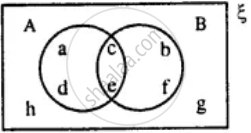# From the Given Diagram Find : (A ∪ B)' - Mathematics

Diagram

From the given diagram find :
(A ∪ B)'#### Solution

A ∪ B = {a, b, c, d, e, f}
∴ (A ∪ B)' = {h, g}

Concept: Venn Diagram
Is there an error in this question or solution?

#### APPEARS IN

Selina Concise Mathematics Class 8 ICSE
Chapter 6 Sets
Exercise 6 (E) | Q 1.5 | Page 75